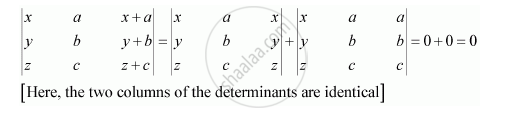Share

# Using the Property of Determinants and Without Expanding, Prove That: |(X, A, X+A),(Y,B,Y+B),(Z,C, Z+ C)| = 0 - CBSE (Commerce) Class 12 - Mathematics

ConceptDeterminant of a Matrix of Order 3 × 3

#### Question

Using the property of determinants and without expanding, prove that:

|(x, a, x+a),(y,b,y+b),(z,c, z+ c)| = 0

#### SolutionIs there an error in this question or solution?

#### Video TutorialsVIEW ALL 

Solution Using the Property of Determinants and Without Expanding, Prove That: |(X, A, X+A),(Y,B,Y+B),(Z,C, Z+ C)| = 0 Concept: Determinant of a Matrix of Order 3 × 3.
S